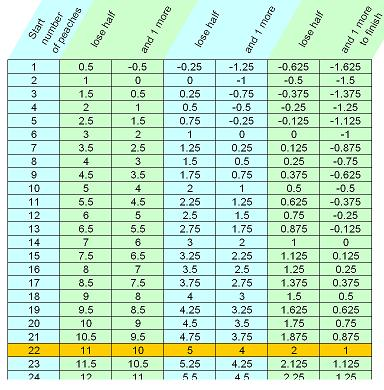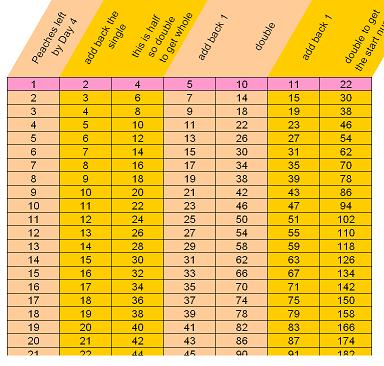#### You may also like### Triangle Incircle Iteration

Keep constructing triangles in the incircle of the previous triangle. What happens?### Loopy

Investigate sequences given by $a_n = \frac{1+a_{n-1}}{a_{n-2}}$ for different choices of the first two terms. Make a conjecture about the behaviour of these sequences. Can you prove your conjecture?### Converging Means

Take any two positive numbers. Calculate the arithmetic and geometric means. Repeat the calculations to generate a sequence of arithmetic means and geometric means. Make a note of what happens to the two sequences.

# Peaches in General

##### Age 14 to 16 Challenge Level:

Here's a spreadsheet file that helps - Peaches Generally

There are three pages or sheets in this file (look for the coloured tabs at the bottom)

The first called 'Forwards' shows the basic Peaches problem and the solution of 22 is picked out in yellow.The second sheet is called 'Backwards' which starts with the end number of peaches and works back to the start number.

This is more efficient. Can you see why ?The third sheet is called 'with Variables' and will help you generalize.

The general process is to have repetitions of two alternating actions. One action is to multiply by a constant amount. In the basic Peaches problem, that multiplier is 0.5, when half the peaches remain. The second action is to add, or subtract, a constant amount. That value is -1 in the original setup.

Experiment varying these two values. You should quickly find some patterns to think about.

What's going on, and why does it happen ?

You may also notice that there isn't really a 'forwards' or 'backwards', just a line of values and a repeated pair of actions which takes you along the line in the direction you choose. Choosing the opposite direction just uses the inverses of those actions and in the opposite order.

#### Once you have a really good feel for this you might like to find out about Repayment Mortgages.

There's a multiplier, involving the interest rate, and a constant addition, the amount of the loan paid off each month.
That addition is negative because the loan amount, which is the quantity we really care about, is reduced rather tha increased by each monthly payment.

And if you do get that far you'll have seen something very important about mathematics. A monkey eating peaches might seem a rather childish puzzle, but once we start to generalize the problem we find a valuable abstract form which will appear in the most surprising places.

A mathematician's task is to have a good stock of these abstract forms and to know their key properties.

Mathematicians are always looking for new forms and new properties; posing questions around simple situations is a great way to make these discoveries.